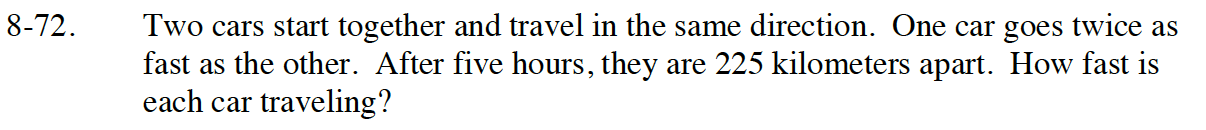### Home > CC4 > Chapter 8 > Lesson 8.1.5 > Problem8-72

8-72.

Two cars start together and travel in the same direction. One car goes twice as fast as the other. After five hours, they are 225 kilometers apart. How fast is each car traveling? Homework Help ✎Remember d = r t. Draw a picture to visualize the situation.

Equation for car 1: d = r (5) where r is the rate and 5 hours is the time.

Equation for car 2: d = 2r (5), where 2r is twice the rate and 5 hours is still the time.

distance of car 1 + distance of car 2 = 225. Solve for r.